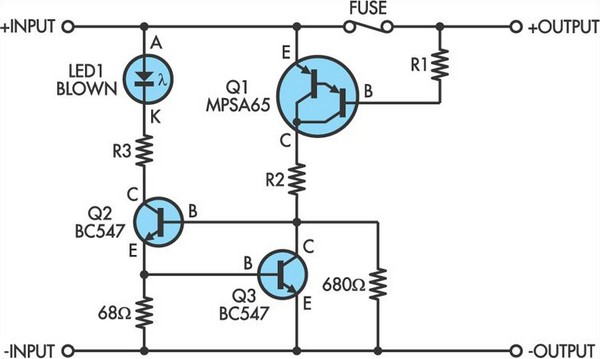# Learning Electronics

Learn to build electronic circuits

# Blown Fuse Indicator

This blown fuse indicator will work with a wide range of DC supply voltages from 5V to 50V. It illuminates LED1 when the fuse blows. With the fuse intact, Q1 is held off and there is no bias current available for the base of Q2. So the LED is off. When the fuse blows, a small current flows via the base-emitter junctions of Darlington transistor Q1, through its base resistor R1 and then via the load. Typically this current will be around 20μA and this turns on Q1 which provides base current to Q2 which then turns on to illuminate the LED.

Circuit diagram:Blown Fuse Indicator Circuit Diagram

The emitter current of Q2 is limited by Q3 which turns when the current reaches about 10mA, to shunt base current away from Q2. The three resistor values not given in the circuit are dependent on the supply voltage and can be calculated from the following simple equations:
• R1(kΩ) = V(DC)/0.02 = 560kΩ for 12V DC
• R2(kΩ) = V(DC)/2 = 5.6kΩ for 12V DC
• R3(Ω) = V(DC)/0.02 = 560Ω for 12V DC
R3 should be included for voltages above about 20V otherwise the heat dissipation in Q2 will be too great. At lower voltages it can be omitted. Any general purpose NPN transistors can be used for Q2 and Q3, provided they will handle the DC supply voltage. The PNP Darlington, Q1, could be an MPSA65, available from Dick Smith Electronics (Cat Z-2088).
Author: Keith Gooley - Copyright: Silicon Chip